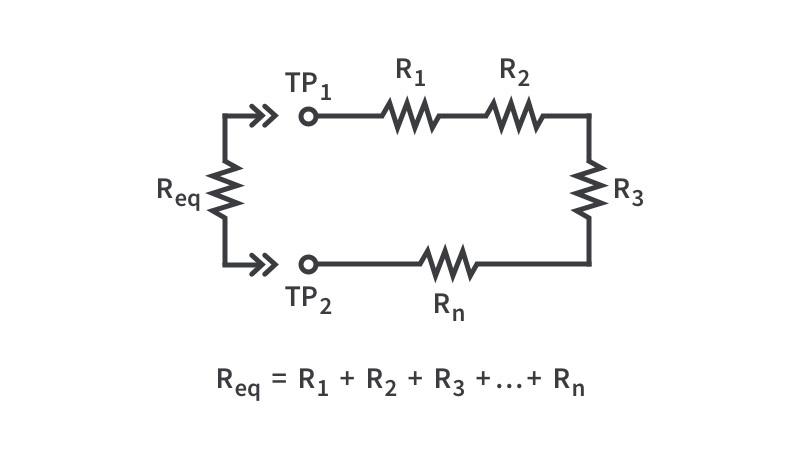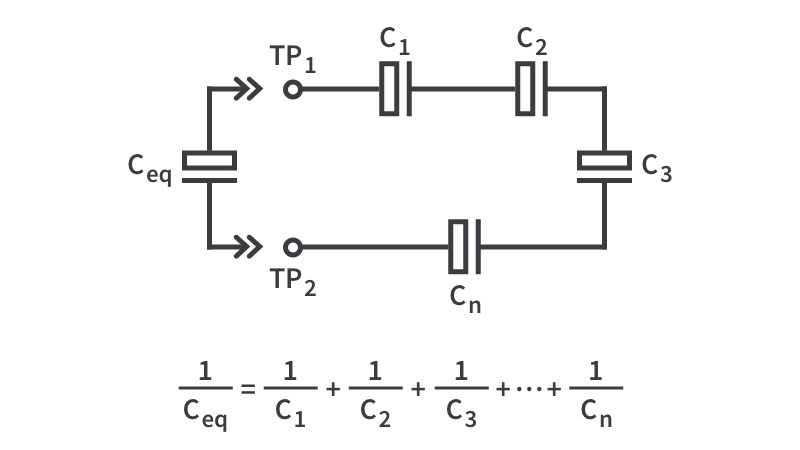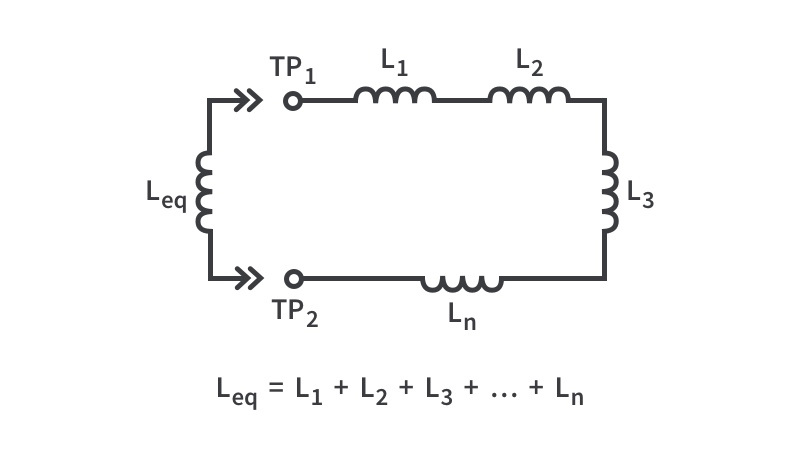# Series EquivalenceInputOutputCalculating the series equivalence of resistors is quite straightforward as you simply sum the resistance of all the resistors together. Double-checking your work is always good, so we’ve provided this “Series Equivalence for Resistors” calculator to make your life even better.InputOutputSeries equivalence of a capacitor is calculated the same way that parallel equivalence is calculated for resistors and inductors. The math isn’t the most difficult you’ll face doing electrical or electronics engineering but still, we’re all human and can make mistakes. Check out the equation above to do this manually, or use the calculator - either way, we’re sure you’ll do great.InputOutput